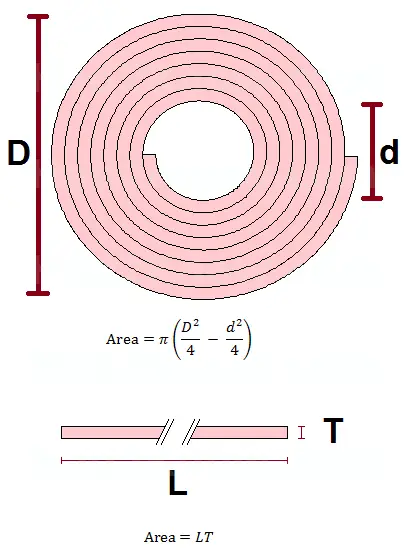# How to Calculate the Length of a Rolled Sheet

Rolled Sheet Calculator
Outer Diameter
D =
Inner Diameter
d =
Thickness
T =

When a sheet of pliable material is coiled it forms the shape of a hollow cylinder. If you know the dimensions of the cylinder and the thickness of the material, you can use simple geometric formulas to calculate the total length of the rolled sheet.

(And conversely if you know the cylinder's measurements and the length of the material, you can compute the thickness of the material. See the companion article.)

The cross-sectional shape of the roll is an annulus. If the material is laid flat, the cross-sectional shape is a long skinny rectangle. The areas of these two shapes must be equal, and you can use this fact to solve for the length.

See image below:If the outer diameter of the rolled material is D, the inner diameter d, the thickness of the material T, and the length L, then the following equation is true:

π[D2/4 - d2/4] = LT

The quantity on the left is the area of the annulus and the quantity on the right is the area of the rectangle. To solve for L, you simply divide both sides by T:

π[D2/4 - d2/4]/T = L

This formula gives the length of the rolled sheet in terms of the cylinder's outer diameter, inner diameter, and the material's thickness. Remember to use the same units for each variable.

### Example

Suppose a cylindrical roll is 230 mm in total diameter, with an inner diameter of 65 mm. If the material is 0.4 mm thick, what is the length of the material?

Since D = 230, d = 65, and T = 0.4, you can compute L with the equation above:

L = π[D2/4 - d2/4]/T
= π[2302/4 - 652/4]/0.4
= π[12168.75]/0.4
= 95573.139 mm, or 95.6 meters.